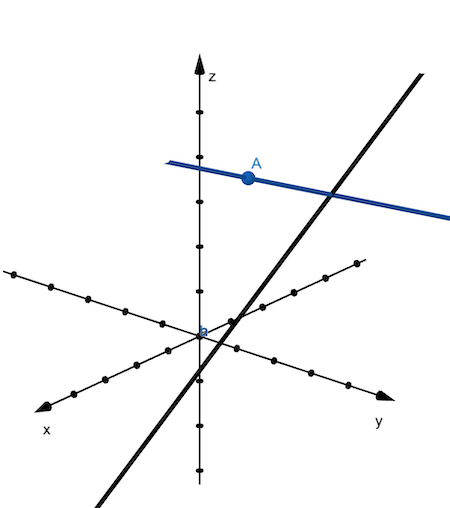# Find parametric equations for the line through the point (2, 3, 5) that is perpendicular to the...

## Question:

Find parametric equations for the line through the point {eq}\displaystyle (2,\ 3,\ 5) {/eq} that is perpendicular to the line {eq}x = 1 - t,\ y = 1 + t,\ z = 2 t {/eq} and intersects this line. Use Maple to plot the two lines on the same plot.

## Planes in 3D

To obtain the line passing through a given point and perpendicular on a given line and intersecting it,

we will obtain the tangent plane containing the given point with the normal vector given by the given line.

To find the line in the plane that intersect the normal line, we will obtain the point of intersection of the plane with the normal line.

To find the line through {eq}\displaystyle (2,3,5) {/eq} perpendicular to the line {eq}\displaystyle x=1-t, y=1+t, z=2t, {/eq} that intersects this line,

we will first, find the plane going through the given point and perpendicular to the given line.

The plane has the normal vector {eq}\displaystyle \langle -1,1,2\rangle {/eq}

so, the plane equation is {eq}\displaystyle -(x-2)+(y-3)+2(z-5)=0. {/eq}

To find the line in this plane that intersects the given line, we will find the point of intersection of the given line with the plane,

which is given by solving {eq}\displaystyle x=1-t, y=1+t, z=2t \text{ and }-(x-2)+(y-3)+2(z-5)=0 \implies -1+t +2 +1+t-3+2(2t-5)=0\implies t=\frac{11}{6} \\ \text{ and the Cartesian coordinates are } x=-\frac{5}{6}, y=\frac{17}{6}, z=\frac{11}{3}. {/eq}

Therefore the line that passes through {eq}\displaystyle (2,3,5) \text{ and } \left(-\frac{5}{6}, \frac{17}{6}, \frac{11}{3}\right) {/eq}

is the line perpendicular and intersecting the given parametric line.

The line passing through the above two points is {eq}\displaystyle x= 2+\left(-\frac{5}{6}-2\right)\ t, y=3+\left(\frac{17}{6}-3\right)\ t, z=5+\left( \frac{11}{3}-5\right)\ t, t\in\mathbf{R}\iff\\ \displaystyle \boxed{x= 2-\frac{17 t}{6}, y=3-\frac{ t}{6}, z=5- \frac{4 t}{3}, t\in\mathbf{R}}. {/eq}

Below, we plotted the given line with black solid line, the given point, shown as A and the line (obtained above) passing through A and perpendicular on the black line, with blue solid line.